# 论文引入

• 注意模块通过基于辅助分类器获得的注意力图区分源域和目标域，帮助模型知道在何处进行密集转换。

# 模型结构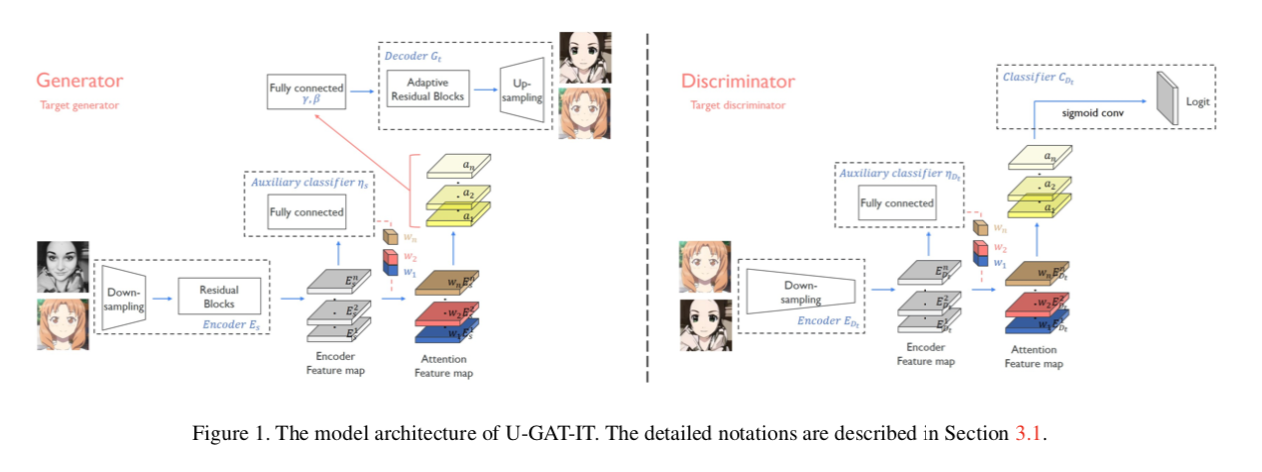## CAM & Auxillary classifier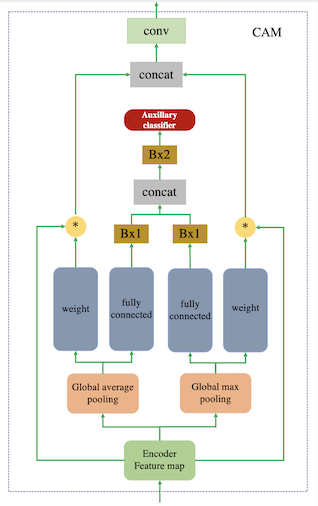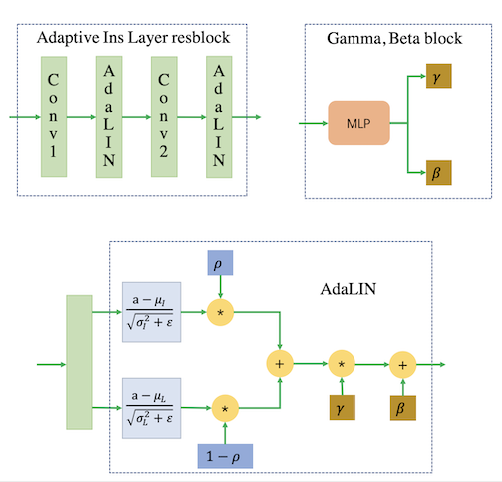## 损失函数

def adaptive_instance_layer_norm(x, gamma, beta, smoothing=True, scope='instance_layer_norm'):
with tf.variable_scope(scope):
ch = x.shape[-1]
eps = 1e-5
# 计算Instance mean，sigma and ins
ins_mean, ins_sigma = tf.nn.moments(x, axes=[1, 2], keep_dims=True)
x_ins = (x - ins_mean) / (tf.sqrt(ins_sigma + eps))

# 计算Layer mean，sigma and ln
ln_mean, ln_sigma = tf.nn.moments(x, axes=[1, 2, 3], keep_dims=True)
x_ln = (x - ln_mean) / (tf.sqrt(ln_sigma + eps))

# 给定rho的范围，smoothing控制rho的弹性范围
if smoothing:
rho = tf.get_variable("rho", [ch], initializer=tf.constant_initializer(0.9),
constraint=lambda x: tf.clip_by_value(x,
clip_value_min=0.0, clip_value_max=0.9))
else:
rho = tf.get_variable("rho", [ch], initializer=tf.constant_initializer(1.0),
constraint=lambda x: tf.clip_by_value(x,
clip_value_min=0.0, clip_value_max=1.0))

# rho = tf.clip_by_value(rho - tf.constant(0.1), 0.0, 1.0)

x_hat = rho * x_ins + (1 - rho) * x_ln

x_hat = x_hat * gamma + beta

return x_hat


# 实验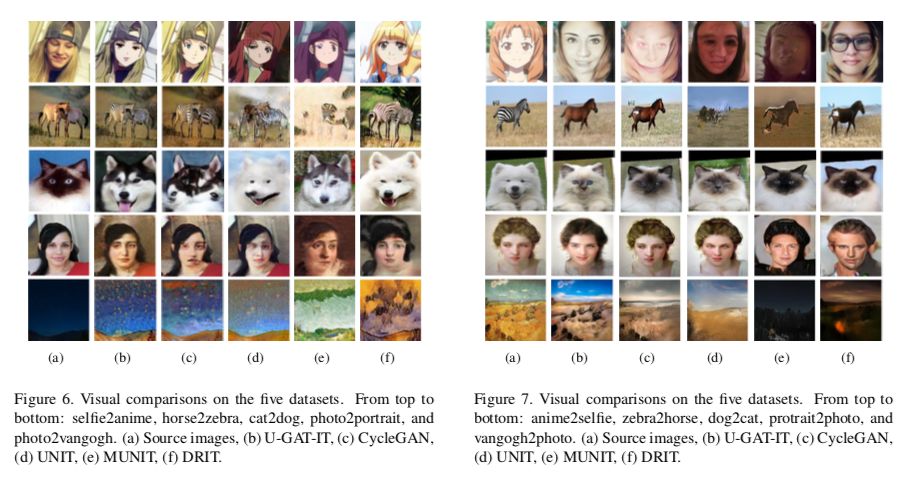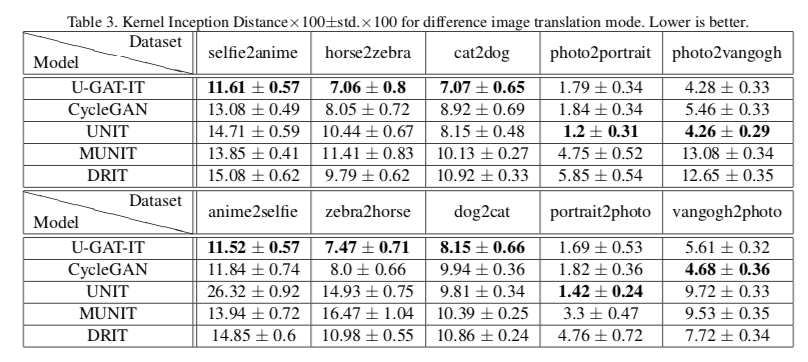# 参考文献

 Goodfellow I, Pouget-Abadie J, Mirza M, et al. Generative adversarial nets[C]//Advances in neural information processing systems. 2014: 2672-2680.

 B. Zhou, A. Khosla, A. Lapedriza, A. Oliva, and A. Torralba. Learning deep features for discriminative localization. In Computer Vision and Pattern Recognition (CVPR), 2016 IEEE Conference on, pages 2921–2929. IEEE, 2016. 2, 3

 H. Nam and H.-E. Kim. Batch-instance normalization for adaptively style-invariant neural networks. arXiv preprint arXiv:1805.07925, 2018. 2, 3